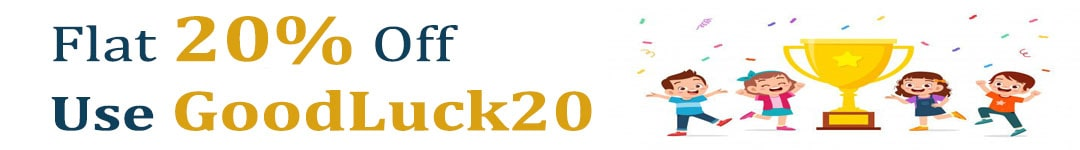International Maths Olympiad Forum By SOF Olympiad Trainer - Page 455

# User ForumSubject :IMO    Class : Class 8

## Ans 1: (Master Answer)

Class : Class 1
The correct answer is D.

Subject :IMO    Class : Class 3

## Ans 1: (Master Answer)

Class : Class 1
The correct answer is A.

Class : Class 4

## Ans 3:

Class : Class 2

Subject :IMO    Class : Class 8

## Ans 1:

Class : Class 8

Subject :IMO    Class : Class 8

## Ans 1:

Class : Class 8
Answer is C. a^2 ÷ 2 Because diagonals of a square are equal. And area of rhombus formula can be used here because both have all sides equal. Area of rhombus= 1/2(diagonal 1 × diagonal 2) Because diagonals are equal, we write it as : a × a÷ 2 =a^2 ÷2

Subject :IMO    Class : Class 6

## Ans 1:

Class :
B: New number is smaller than original number.

Class : Class 8
option B

## Ans 3:

Class : Class 6
option B

Subject :IMO    Class : Class 4

Class : Class 4
The answer is C

## Ans 2:

Class : Class 5
Answer is C as the question says 3098+1427<5420+2018 and we have to find how much less 3098+1427<5420+2018, which is 5420+2018-3098+1427 and to get this we have to follow the BODMAS rule, which is 7438-4525=2913.

Subject :IMO    Class : Class 7

Class : Class 10
Ans is d 20

Class : Class 7
B

## Ans 3:

Class : Class 9
D

Subject :IMO    Class : Class 7

Class : Class 7
D

Class : Class 9
d

## Ans 3:

Class : Class 9
Angle BAC = x + 40 - x => 40 As triangle ABC is an isosceles triangle with AB = AC, angle acb = angle abc = x Therefore, as angle abc + angle acb + angle bac = 180 then, x + x + 40 = 180 =>2x = 180 - 40 =>2x = 140 =>x = 70

Subject :IMO    Class : Class 7

## Ans 1:

Class : Class 8
Since triangle ABD is congruent to triangle ACD by SSS rule, so the altitude will also be the same. Which means BE=EC, as these are the altitudes of the triangles.

Subject :IMO    Class : Class 4

## Ans 1:

Class : Class 4
The answer is b}4789

## Ans 2:

Class : Class 4
Answer is B),how? Because the first line mentions that the forth digit is the greatest digit which is nine so option A) and option C) cannot be the answer. The second line mentions that the first digit is double of the number two so option D) cannot be the answer. So the answer is B)

Class : Class 7
B) 4789